# Civil Engineering - Structural Design Specifications

### Exercise :: Structural Design Specifications - Section 1

6.

For design of a column the eccentricity of loading shall be taken as the distance from the assumed point of application of the load to the centroid of the column, subject to a minimum of

 A. 10 mm B. 20 mm C. 30 mm D. 40 mm E. 50 mm

Explanation:

No answer description available for this question. Let us discuss.

7.

If l is the effective span in metres and D is overall depth of the section in mm, the structure may be accepted after 24 hour load test, provided the maximum deflection in mm is less than

 A.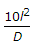B.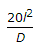C.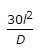D.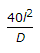Explanation:

No answer description available for this question. Let us discuss.

8.

The approximate value of torsion constant k for a box of depth d, width b, and having uniform thickness t, is

 A.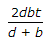B.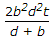C.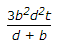D.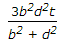Explanation:

No answer description available for this question. Let us discuss.

9.

In the formula a + bk for the calculation of the net effective section of single angles in tension connected by one leg only, the value of k, is

 A.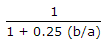B.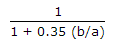C.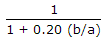D.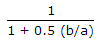Explanation:

No answer description available for this question. Let us discuss.

10.

The concrete is deemed not to comply with the strength requirements if the strength is less than the greater of

 A. characteristic strength minus 1.35 times the standard deviation B. 0.8 times the characteristic strength C. the average strength of all the samples is less than the characteristic strength plus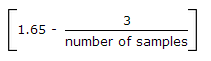times the standard deviation. D. all the above.